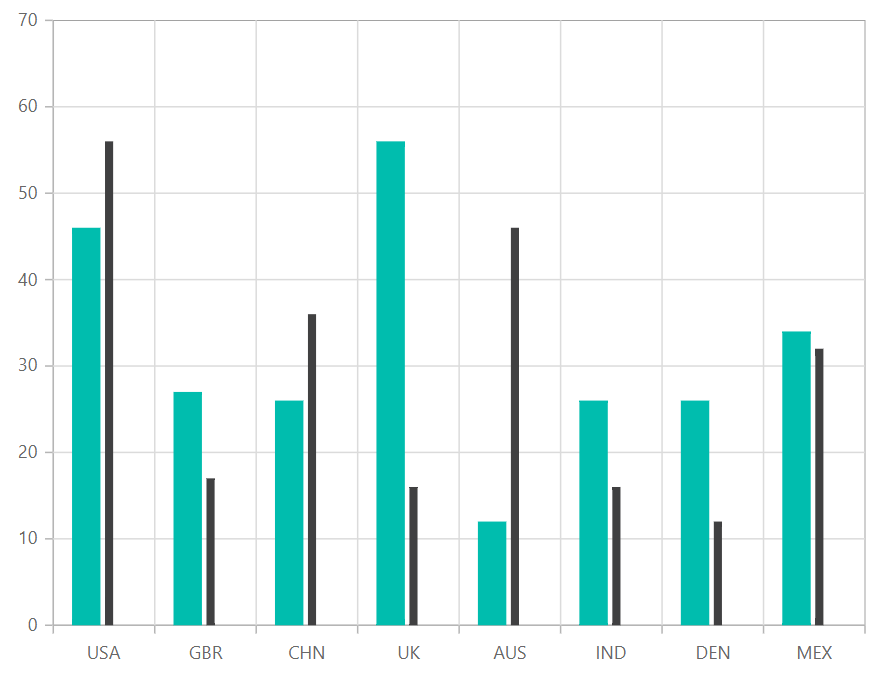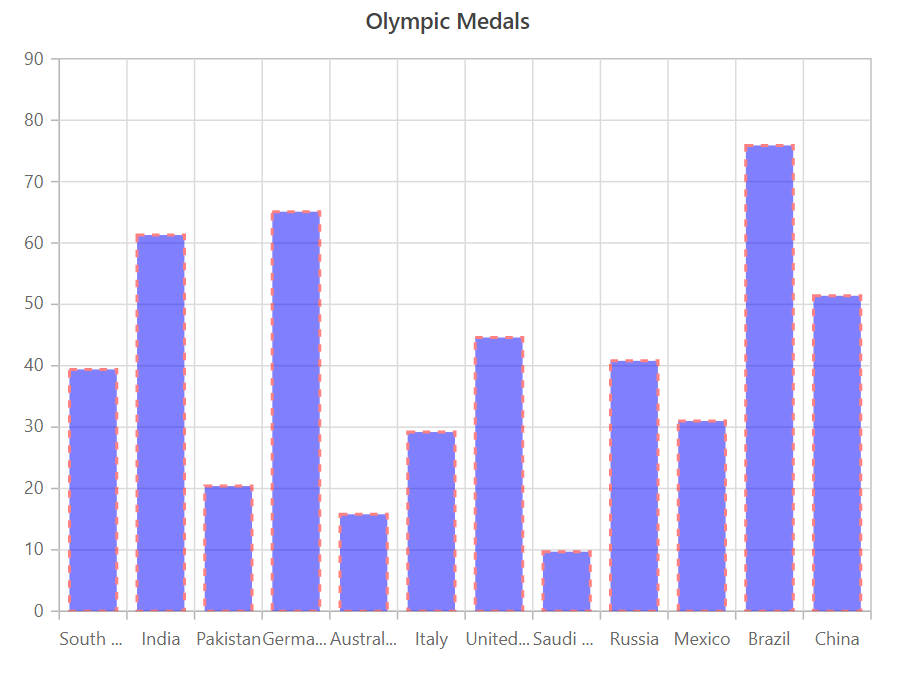# Column Chart in Blazor Charts Component

4 Aug 20215 minutes to read

## Column

Column Chart is the most common chart type that is used to compare Frequency, Count, Total, or Average of data in different categories. It is ideal for showing variations in the value of an item over time. To render a column series, set series Type as Column.

``````@using Syncfusion.Blazor.Charts

<SfChart>
<ChartPrimaryXAxis ValueType="Syncfusion.Blazor.Charts.ValueType.Category"/>

<ChartSeriesCollection>
<ChartSeries DataSource="@MedalDetails" XName="X" YName="Y" Type="ChartSeriesType.Column">
</ChartSeries>
</ChartSeriesCollection>
</SfChart>

@code{
public class ChartData
{
public string X { get; set; }
public double Y { get; set; }
}

public List<ChartData> MedalDetails = new List<ChartData>
{
new ChartData { X= "South Korea", Y= 39.4 },
new ChartData { X= "India", Y= 61.3 },
new ChartData { X= "Pakistan", Y= 20.4 },
new ChartData { X= "Germany", Y= 65.1 },
new ChartData { X= "Australia", Y= 15.8 },
new ChartData { X= "Italy", Y= 29.2 },
new ChartData { X= "United Kingdom", Y= 44.6 },
new ChartData { X= "Saudi Arabia", Y= 9.7 },
new ChartData { X= "Russia", Y= 40.8 },
new ChartData { X= "Mexico", Y= 31 },
new ChartData { X= "Brazil", Y= 75.9 },
new ChartData { X= "China", Y= 51.4 }
};
}``````

Refer to our Blazor Column Charts feature tour page to know about its other groundbreaking feature representations. Explore our Blazor Column Charts Example to compare Frequency, Count, Total, or Average of data in different categories.

## Column Space and Width

The ColumnSpacing and ColumnWidth properties are used to customize the space between columns.

``````@using Syncfusion.Blazor.Charts

<SfChart>
<ChartPrimaryXAxis ValueType="Syncfusion.Blazor.Charts.ValueType.Category" />

<ChartSeriesCollection>
<ChartSeries DataSource="@MedalDetails" XName="X" YName="Y" Type="ChartSeriesType.Column"  ColumnSpacing="0.2" ColumnWidth="0.2" >
</ChartSeries>
</ChartSeriesCollection>
</SfChart>

@code{
public class ChartData
{
public string X { get; set; }
public double Y { get; set; }
}

public List<ChartData> MedalDetails = new List<ChartData>
{
new ChartData { X= "South Korea", Y= 39.4 },
new ChartData { X= "India", Y= 61.3 },
new ChartData { X= "Pakistan", Y= 20.4 },
new ChartData { X= "Germany", Y= 65.1 },
new ChartData { X= "Australia", Y= 15.8 },
new ChartData { X= "Italy", Y= 29.2 },
new ChartData { X= "United Kingdom", Y= 44.6 },
new ChartData { X= "Saudi Arabia", Y= 9.7 },
new ChartData { X= "Russia", Y= 40.8 },
new ChartData { X= "Mexico", Y= 31 },
new ChartData { X= "Brazil", Y= 75.9 },
new ChartData { X= "China", Y= 51.4 }
};
}``````## Series Customization

The following properties can be used to customize the Column series.

``````@using Syncfusion.Blazor.Charts

<SfChart>
<ChartPrimaryXAxis ValueType="Syncfusion.Blazor.Charts.ValueType.Category" />

<ChartSeriesCollection>
<ChartSeries DataSource="@MedalDetails" XName="X" YName="Y" Type="ChartSeriesType.Column" Opacity="0.5"
DashArray="5,5" Fill="blue" >
</ChartSeries>
</ChartSeriesCollection>
</SfChart>

@code{
public class ChartData
{
public string X { get; set; }
public double Y { get; set; }
}

public List<ChartData> MedalDetails = new List<ChartData>
{
new ChartData { X= "South Korea", Y= 39.4 },
new ChartData { X= "India", Y= 61.3 },
new ChartData { X= "Pakistan", Y= 20.4 },
new ChartData { X= "Germany", Y= 65.1 },
new ChartData { X= "Australia", Y= 15.8 },
new ChartData { X= "Italy", Y= 29.2 },
new ChartData { X= "United Kingdom", Y= 44.6 },
new ChartData { X= "Saudi Arabia", Y= 9.7 },
new ChartData { X= "Russia", Y= 40.8 },
new ChartData { X= "Mexico", Y= 31 },
new ChartData { X= "Brazil", Y= 75.9 },
new ChartData { X= "China", Y= 51.4 }
};
}``````Refer to our Blazor Charts feature tour page for its groundbreaking feature representations and also explore our Blazor Chart Example to know various chart types and how to represent time-dependent data, showing trends at equal intervals.